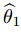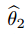# Hausman Test for Endogeneity (Hausman Specification Test)

Regression Analysis > Hausman Test

## What is the Hausman Test?

The Hausman Test (also called the Hausman specification test) detects endogenous regressors (predictor variables) in a regression model. Endogenous variables have values that are determined by other variables in the system. Having endogenous regressors in a model will cause ordinary least squares estimators to fail, as one of the assumptions of OLS is that there is no correlation between an predictor variable and the error term. Instrumental variables estimators can be used as an alternative in this case. However, before you can decide on the best regression method, you first have to figure out if your predictor variables are endogenous. This is what the Hausman test will do.

This test is also called the Durbin–Wu–Hausman (DWH) test or the augmented regression test for endogeneity.

## Use in Panel Data Analysis

The Hausman test is sometimes described as a test for model misspecification. In panel data analysis (the analysis of data over time), the Hausman test can help you to choose between fixed effects model or a random effects model. The null hypothesis is that the preferred model is random effects; The alternate hypothesis is that the model is fixed effects. Essentially, the tests looks to see if there is a correlation between the unique errors and the regressors in the model. The null hypothesis is that there is no correlation between the two.

## Test Results

Interpreting the result from a Hausman test is fairly straightforward: if the p-value is small (less than 0.05), reject the null hypothesis. The problem comes with the fact that many versions of the test — with different hypothesis and possible conclusions — exist. In fact, some of the available tests suggest “…opposite conclusions about the null hypothesis” (Chmelarova, 2007). Check your software and make sure you know which null hypothesis you are actually accepting or rejecting.

One of the more common forms of the test is comparing estimators. For example, in this Stata example, you’re comparingagainst. The null hypothesis is that the estimatoris an efficient (and consistent) estimator of the true population parameters.

## References

Beyer, W. H. CRC Standard Mathematical Tables, 31st ed. Boca Raton, FL: CRC Press, pp. 536 and 571, 2002.
Dodge, Y. (2008). The Concise Encyclopedia of Statistics. Springer.
Kotz, S.; et al., eds. (2006), Encyclopedia of Statistical Sciences, Wiley.
Vogt, W.P. (2005). Dictionary of Statistics & Methodology: A Nontechnical Guide for the Social Sciences. SAGE.

CITE THIS AS:
Stephanie Glen. "Hausman Test for Endogeneity (Hausman Specification Test)" From StatisticsHowTo.com: Elementary Statistics for the rest of us! https://www.statisticshowto.com/hausman-test/